# Thermochemistry Thermochemistry Study of energy changes that occur

• Slides: 16Thermochemistry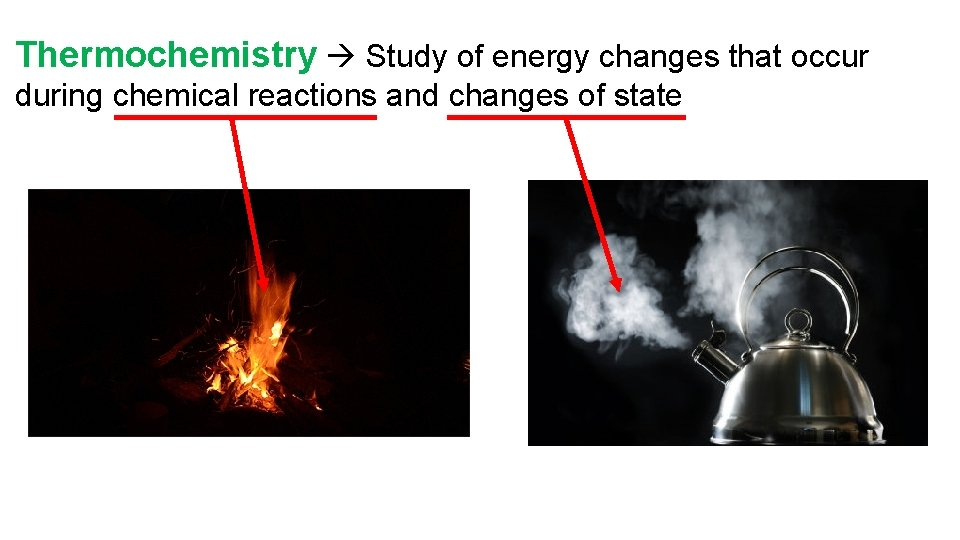Thermochemistry Study of energy changes that occur during chemical reactions and changes of stateEndothermic Process that absorbs/gains heat Heat flows into the system (+q) Heat removed from the surroundings (-q) Surroundings feel cold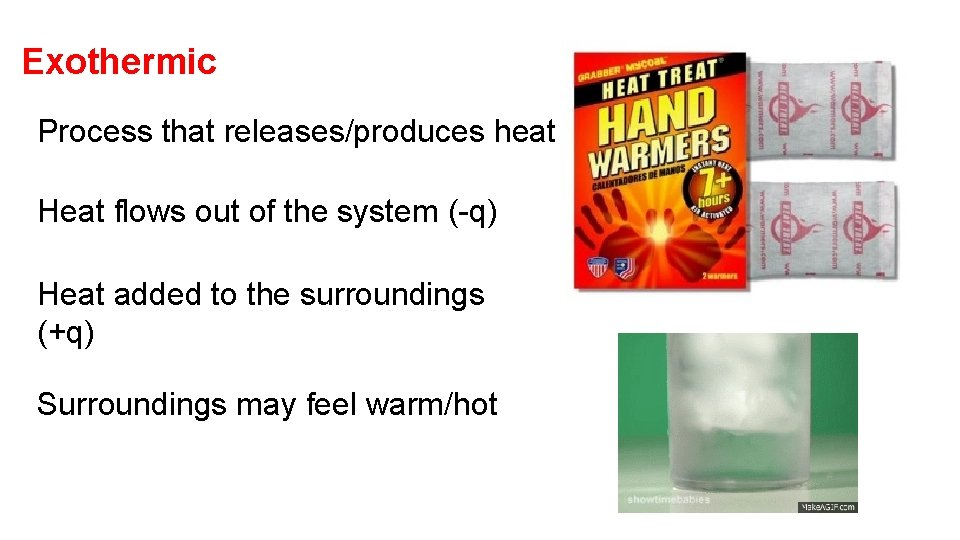Exothermic Process that releases/produces heat Heat flows out of the system (-q) Heat added to the surroundings (+q) Surroundings may feel warm/hotExothermic -q -q Endothermic +q -q +q +qEnthalpy (H) the heat content of a system units for enthalpy are k. J ΔH change in enthalpy Endothermic = + ΔH assuming pressure is constant, ΔH = q Exothermic = - ΔH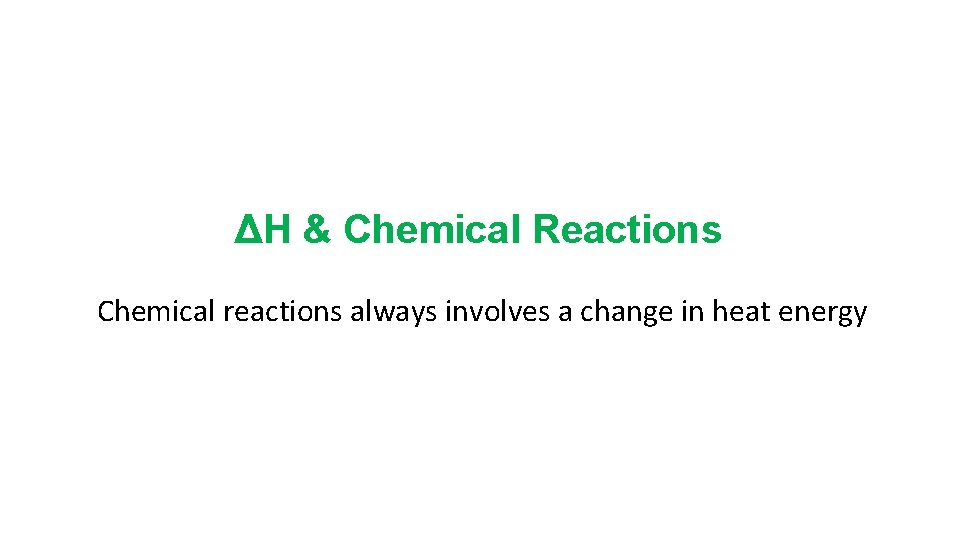ΔH & Chemical Reactions Chemical reactions always involves a change in heat energy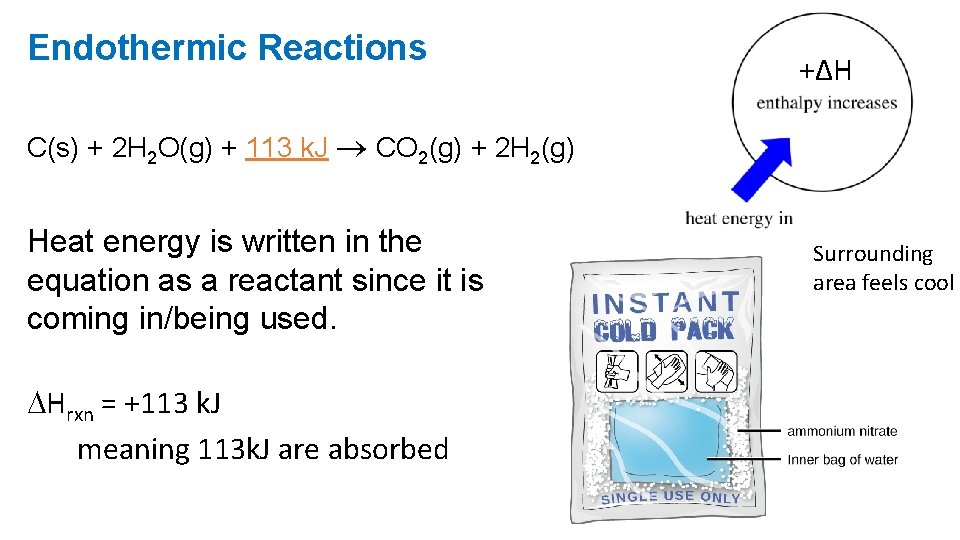Endothermic Reactions +ΔH C(s) + 2 H 2 O(g) + 113 k. J CO 2(g) + 2 H 2(g) Heat energy is written in the equation as a reactant since it is coming in/being used. Hrxn = +113 k. J meaning 113 k. J are absorbed Surrounding area feels coolPotential energy diagram Endothermic because…. 1. Products have more energy than the reactants 2. ΔH is positive Activation Energy DHExothermic Reactions -ΔH C 3 H 8 + 5 O 2 3 CO 2 + 4 H 2 O + 2043 k. J Heat energy is written in the equation as a product since it is released/produced. ΔHrxn = -2043 k. J. . . meaning 2043 k. J of heat is released Surrounding area feels warm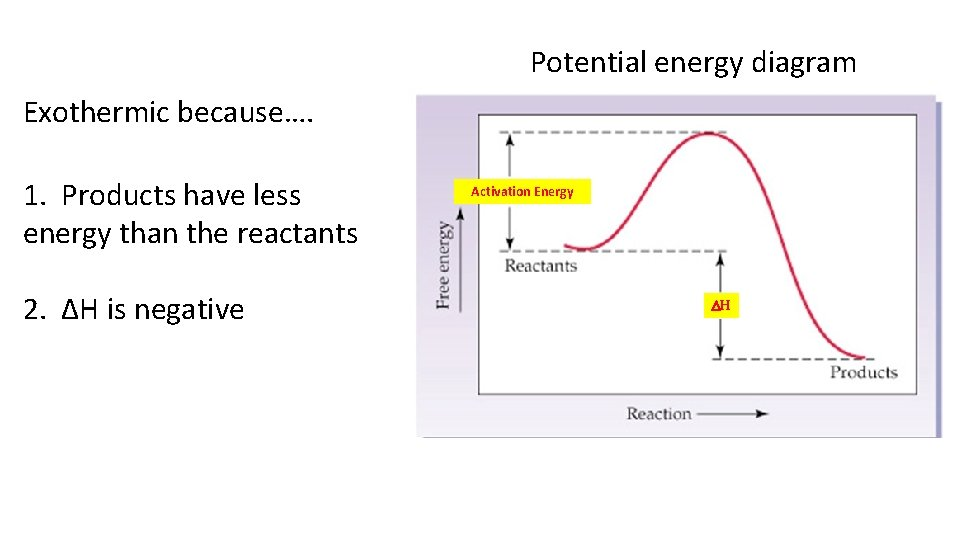Potential energy diagram Exothermic because…. 1. Products have less energy than the reactants 2. ΔH is negative Activation Energy DH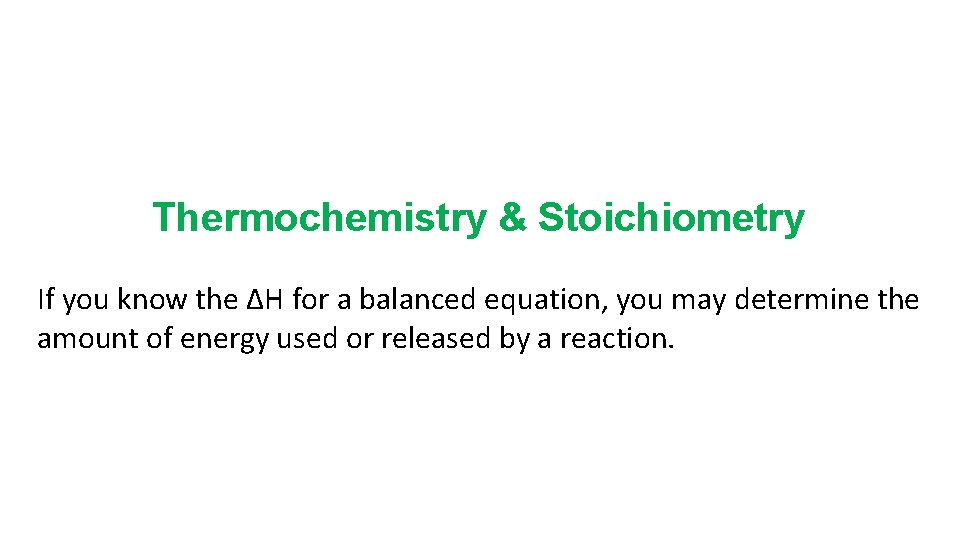Thermochemistry & Stoichiometry If you know the ΔH for a balanced equation, you may determine the amount of energy used or released by a reaction.ΔH (enthalpy) is proportional to the coefficients for a balanced equation, therefore they may be used to write conversion factors. 2 H 2 O 2(l) 2 H 2 O(l) + O 2(g) Hrxn = -190 k. J 2 mole H 2 O 2 = -190 k. J Or 1 mole O 2 = -190 k. J Or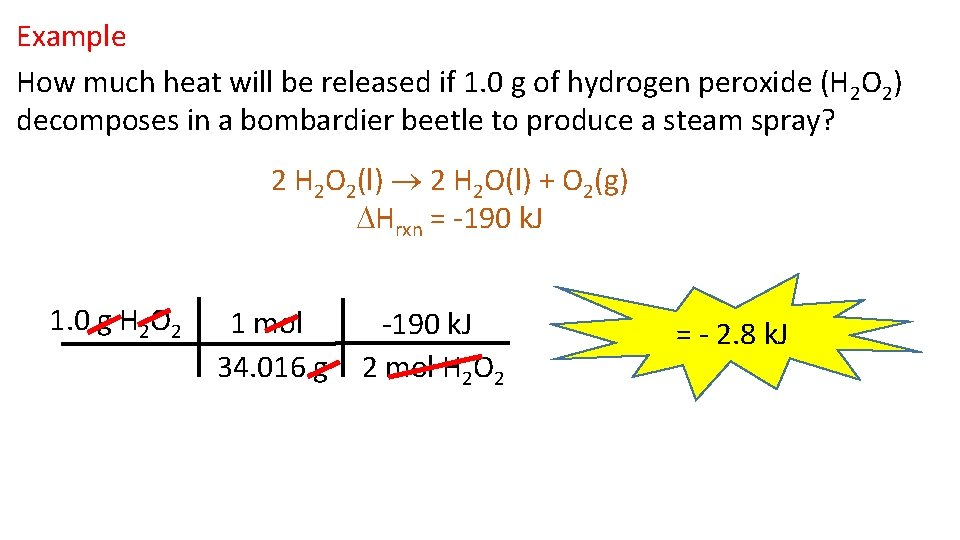Example How much heat will be released if 1. 0 g of hydrogen peroxide (H 2 O 2) decomposes in a bombardier beetle to produce a steam spray? 2 H 2 O 2(l) 2 H 2 O(l) + O 2(g) Hrxn = -190 k. J 1. 0 g H 2 O 2 1 mol 34. 016 g -190 k. J 2 mol H 2 O 2 = - 2. 8 k. J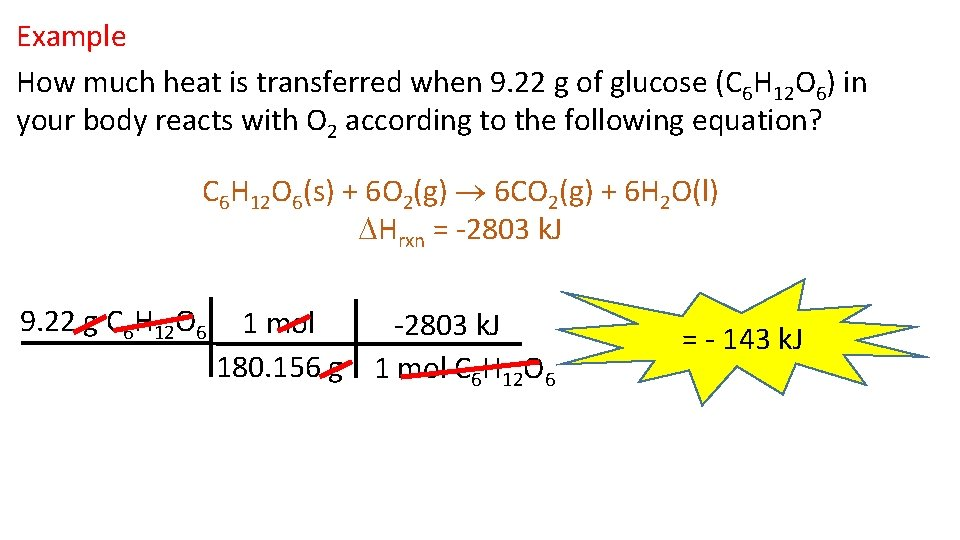Example How much heat is transferred when 9. 22 g of glucose (C 6 H 12 O 6) in your body reacts with O 2 according to the following equation? C 6 H 12 O 6(s) + 6 O 2(g) 6 CO 2(g) + 6 H 2 O(l) Hrxn = -2803 k. J 9. 22 g C 6 H 12 O 6 1 mol -2803 k. J 180. 156 g 1 mol C 6 H 12 O 6 = - 143 k. J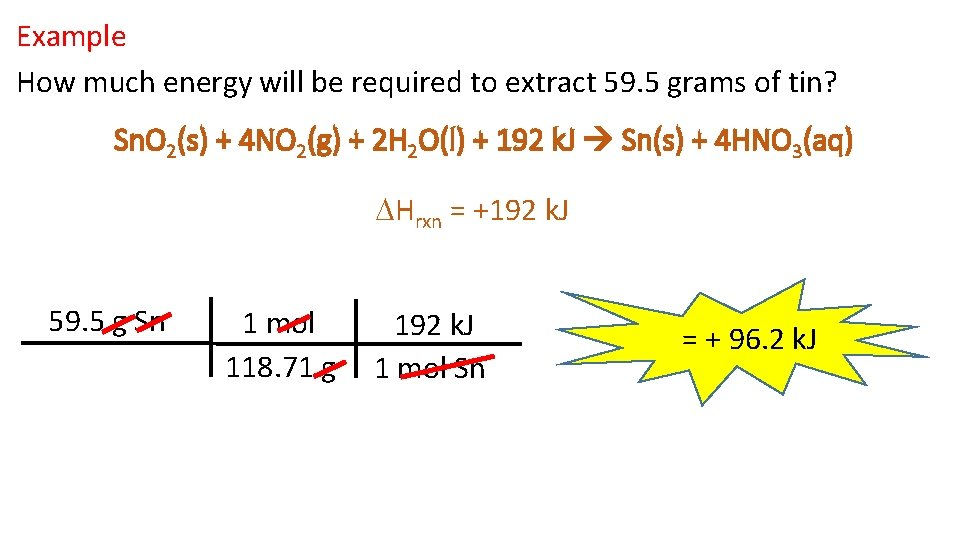Example How much energy will be required to extract 59. 5 grams of tin? Sn. O 2(s) + 4 NO 2(g) + 2 H 2 O(l) + 192 k. J Sn(s) + 4 HNO 3(aq) Hrxn = +192 k. J 59. 5 g Sn 1 mol 118. 71 g 192 k. J 1 mol Sn = + 96. 2 k. J